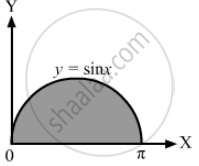Department of Pre-University Education, KarnatakaPUC Karnataka Science Class 11

# Find the Area Enclosed by the Curve Y = Sin X and the X-axis Between X = 0 and X = π. - Physics

Sum

Find the area enclosed by the curve y = sin x and the X-axis between x = 0 and x = π.

#### Solution

The given equation of the curve is y = sin x.The required area can found by integrating y w.r.t x within the proper limits.

$\therefore \text{Area} = \int_{x_1}^{x_2} \text{ y }dx = \int_0^\pi \sin \text{ x } dx$

$= \left[ - \cos x \right]_0^\pi$

$= - \cos \pi - \left( - \cos 0 \right)$

= 1 + 1 = 2 sq. unit

Concept: Physics Related to Technology and Society
Is there an error in this question or solution?

#### APPEARS IN

HC Verma Class 11, Class 12 Concepts of Physics Vol. 1
Chapter 2 Physics and Mathematics
Exercise | Q 25 | Page 30

Share